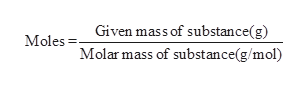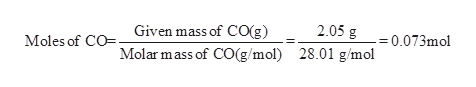How many moles of carbon monoxide are present in 2.05 grams of this compound? _____molesHow many grams of carbon monoxide are present in 2.79 moles of this compound? _____grams

Question
1. How many moles of carbon monoxide are present in 2.05 grams of this compound? _____moles
2. How many grams of carbon monoxide are present in 2.79 moles of this compound? _____grams
Step 1

The moles of a substance is calculated from the given mass of the substance using the below mentioned formula.help_outlineImage TranscriptioncloseGiven mass of substance(g) Moles Molar mass of substance(g/mol) fullscreen
Step 2

The number of moles of carbon monoxide are pr...help_outlineImage TranscriptioncloseMoles of CO Given mass of CO(g) Molar mass of CO(g/mol) 2.05g 0.073mol 28.01 g/mol fullscreen

Want to see the full answer?

See Solution

Want to see this answer and more?

Our solutions are written by experts, many with advanced degrees, and available 24/7

See Solution
Tagged in

General Chemistry